Skip to main content

# A routing algorithm for region division based on virtual spider web model

## Abstract

A disjoint multipath routing algorithm based on region division is proposed to resolve problems such as potential “hot area” around the sink node and path instability caused by frequent movement of routing nodes in the mobile wireless sensor network. This paper first improves the regional division model of the whole network by learning from the predation of spiders, and increases the number of nodes in the inner area by enlarging the width of the inner ring to alleviate the “hot area” problem. Then, three modes based on distance priority, angle priority, and weight priority are proposed to ensure the independence between the paths when generating the disjoint multipath of the mobile node in a certain divided area. Meanwhile, the method of selecting “borrowing node” with minimum weights is adopted to ensure the effectiveness of the path and deal with the path link break problem. Simulation experiments show that the proposed algorithm has a lower packet loss rate and path failure rate than EM-GMR and AGEM algorithms, which effectively prolongs the lifetime of the entire network and is more applicable to networks with dense nodes.

## Introduction

More and more heed has been paid to the research of wireless sensor networks, which is organized by abundant micro sensor nodes deployed in the observation environment through wireless communication. Generally, the sensor nodes are deployed in an unattended environment, while the nodes in the network under the influence of external conditions are highly vulnerable, so that node failure and link break often arise. The multipath routing is one main part of the research on routing fault tolerance, which can scatter data to be transmitted into multiple paths and then proceed to transmit in parallel, so as to reduce delay, balance network load, and lower packet loss rate.

In recent years, many merits of the multipath routing algorithm have drawn the attention of scholars. However, the increase of path number has rapidly added the expense of path creation and maintenance and the complexity of data traffic distribution . Most of the existing multipath routing algorithm researches focus on the clustering routing protocol [2,3,4]. The cluster head nodes are generally fixed in position and have higher energy and a longer communication radius, each of which is in charge of the node movement within the cluster. A multipath routing mechanism based on location information for mobile wireless sensor network is proposed in . When selecting the next-hop node, the algorithm fully considers the residual energy, distance, and mobility factors of neighbor nodes and adopts the fuzzy logic system to determine the next hop. The paper in  selects the node with the minimum mobility and the maximum residual energy as the cluster head through a mobility-based cluster head election algorithm and an adaptive algorithm, which is vastly superior to the mobile protocol in transmission performance and network lifetime. Although this method can improve the energy utilization of the network, each cluster head node not only takes charge of information collection and data fusion, but also transmits information transmitted by other nodes. As a result, the amount of information transmitted by the node closer to the sink node is larger, the energy consumption is higher, the node close to the sink node is extremely easy to die due to excessive energy consumption, and a “hot area” is prone to develop around the sink node. Focusing on this problem, many researchers have proposed an uneven clustering routing algorithm for wireless sensor network and its corresponding improved algorithms, including the uneven clustering routing algorithm based on competition for a cluster head proposed in , the routing algorithm for data fusion based on multi-hop clustering proposed in [8, 9], and the uneven clustering routing algorithm for cluster head selection based on optimal hop number proposed in [10, 11]. To some extent, these algorithms balance the energy consumption of each node in the network, prolong the network lifetime, and reduce the problems of the “hot area” [12, 13]. However, frequent mobility of the nodes breaks the original path and new route discovery causes the instability of the entire network. Therefore, this paper proposes a disjoint multipath routing algorithm based on region division (DMRRD) by learning from the predation of spiders to solve the problem of path instability in the network with mobile nodes, which effectively solves the problems of path failure and “hot area” caused by node mobility.

The rest of the paper is organized as follows. In Section 2, we briefly introduce the virtual spider web model. In Section 3, we propose a disjoint multipath routing and a node movement processing and path repair mechanism, and simulation results and analysis on the performance of a disjoint multipath routing algorithm based on a virtual spider web model. Finally, our main conclusions are drawn in Section 4.

## Methodology

It is assumed that the application scenario of the wireless sensor networks (WSN) is a circular network with only one sink node, where the sink node is on its center without mobility, and other nodes are randomly distributed in the circular region with certain mobility. Each node in the network obtains the location information of itself and neighbor nodes through the built-in GPS device or positioning system.

### Spider web model

Spiders prey by their webs. No matter in which part of the web the spider is, it can reach the prey through different paths, and convey it to the central node. In the left diagram of Fig. 1, the spider can take three paths, each of which does not intersect. In the WSN, it is impossible to deploy the nodes on the spider silk. Most of the nodes in the network are randomly deployed within a certain domain. According to the right diagram in Fig. 1, the nodes in the small region divided by the spider silks are not repeated, so the paths formed by selecting nodes in the different regions are disjoint .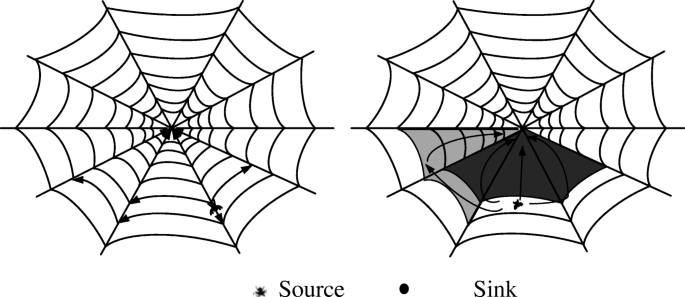Fig. 1

If the spider web above is directly applied to the wireless sensor network, there will be two problems:

(1) Since the region near the center of the spider web has a small area and relatively few nodes, the data groupings that the nodes need to transmit are more than the data transmitted in other regions. The data groupings reaching the sink node need to pass through the nodes in the region, which will result in excessive energy consumption. Consequently, the nodes in these regions are easiest to fail, resulting in a “hot area.”

(2) Since the transverse width of the outermost region of the spider web is relatively large, if it is more than three times the communication radius, it is likely that transverse adjacent regions cannot communicate directly.

### Creation of the virtual spider web model

For the above problems, the spider web in Fig. 1 has been improved and the virtual spider web in Fig. 2a has been created to make the region division of the whole network more reasonable. It is supposed that all the sensor nodes in the WSN have the same communication radius r, the radius of the innermost small circle of the sink node is set as the communication radius between nodes, and the width of the adjacent ring is also r. The width of remaining rings is one half of the communication radius, namely, 0.5 r. Meanwhile, it is necessary to judge whether the subdivision should be done according to the size of the sector arc. When the chord length of the sector is quite larger than the communication radius, it is likely that the next-hop node cannot reach the nodes in the left and right regions, resulting in path reduction, so the small regions in the outer ring are subdivided.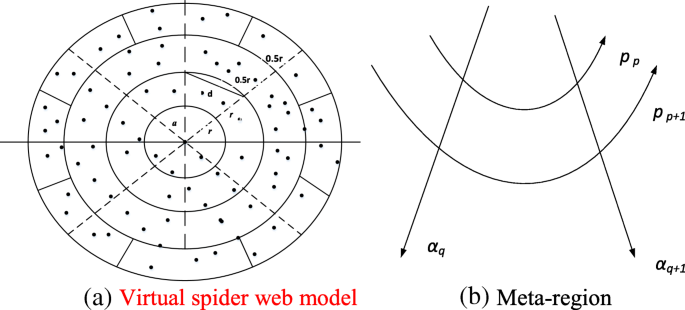Fig. 2

### Definition 1.

Radial line: It refers to a line that extends from a center node in the virtual spider web.

### Definition 2.

Chord line: It refers to a line segment connecting any two points on the virtual spider web.

### Definition 3.

Meta-region: It refers to the inseparable minimum closed region formed by radials and chords in the virtual spider web, as shown in Fig. 2b.

### Definition 4.

Non-overlapping meta-region chains: It refers to a sequence consisting of a series of non-repeating meta-regions, and the meta-region at the end of the sequence contains the center node.

In order to facilitate the mathematical description of the meta-region, the communication radius of the node is set as r. The non-overlapping meta-region chain in the virtual spider web is shown in Fig. 3. Taking sink node A as the center of the polar coordinates and ρ as the radius, a polar coordinate system can be created. The counterclockwise direction is set as the positive direction of the polar angle. If the location of a node is (x, y), it can be converted into the corresponding polar coordinates by formulas (1) and (2) below:

$$\rho =\sqrt{x^2+{y}^2}$$
(1)
$$\alpha =\arctan \frac{y}{x}$$
(2)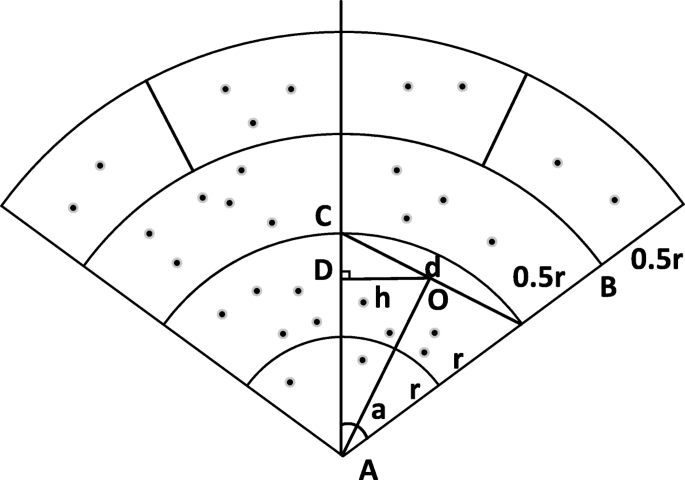Fig. 3

Assuming an isosceles triangle ABC in arbitrary Meta-region of a partitioned domain chain without overlapping elements, BC refers to the chord length of the sector, the apex angle refers to the polar angle, and the length of AB, one of the two equal sides, is L, the side length of the chord BC is d, shown as formula (3):

$$d=2\mathrm{AB}\times \sin \frac{\alpha }{2}=2L\times \sin \frac{\alpha }{2}$$
(3)

Draw a line through point O, which is perpendicular to AC; if the foot point is D, the length h of the perpendicular OD can be calculated based on triangle-related theorems, shown as formula (4):

$$h=\frac{d}{2}\cos \frac{\alpha }{2}=\mathrm{ABsin}\frac{\alpha }{2}\cos \frac{\alpha }{2}=\frac{L}{2}\sin \alpha$$
(4)

According to formula (4), when the angle α is definite, the length of h is positively correlated with the length L of AB. According to the data transmission characteristics of the nodes in the mobile sensor network (if the transverse width is more than three times the communication radius, it is likely that transverse adjacent regions cannot communicate directly); therefore, when h < r, the radius of the two inner rings near the center is expanded to r, and the number of nodes in the inner region is increased, so as to alleviate the problem of a “hot area,” and the radius of the remaining outer rings is set as 0.5 r.

When h ≥ r, the node at point O cannot search for the next-hop node in the left and right regions, decreasing the available multipath. Each region is subdivided into two identical parts to avoid the decrease of the multipath.

### Theorem 1:

In the virtual spider web, it is known that ρp is the left boundary of the polar radius of the meta-region, ρp + 1 is the right boundary of the polar radius of the meta-region, αq is the upper boundary of the polar angle of the meta-region, and αq + 1 is the bottom boundary of the polar angle of the meta-region and the polar coordinates of the source node Si is (ρi, αi); if the meta-region is not subdivided, (ρp, ρp + 1, αq, αq + 1) refers to the corresponding meta-region and there is:

$$\left\{\begin{array}{c}{\rho}_{\mathrm{p}}={\rho}_x\mid {\rho}_x\le {\rho}_i\le {\rho}_{x+1};0\le x<k\ \\ {}{\alpha}_{\mathrm{q}}={\alpha}_x\mid {\alpha}_x\le {\alpha}_i\le {\alpha}_{x+1};0\le x<k\ \end{array}\right.$$
(5)

### Theorem 2:

In the virtual spider web, if the meta-region is subdivided, the radial and chord $$\left({\rho}_q,{\rho}_{q+1},{\theta}_{p^{\prime \prime }},{\theta}_{p^{\prime }+1}\right)$$ refers to the corresponding region.

### Expression of non-overlapping meta-region chain

In the virtual spider model of mobile wireless sensor network, one meta-region A is selected, and the meta-regions B, C, D, E, F, G, and M and an adjacent meta-region A are respectively the middle upper (MU) region, middle down (MD) region, left middle (LM) region, right middle (RM) region, left upper (LU) region, right upper (RU) region, left down (LD) region, and right down (RD) region, eight non-overlapping regions in total. In the current meta-region A, taking a random source node s as the chain head node and L as the sink node, eight non-overlapping meta-region chains can be theoretically created. Three non-overlapping meta-region chains starting from MU, RU, and RM are shown in Fig. 4.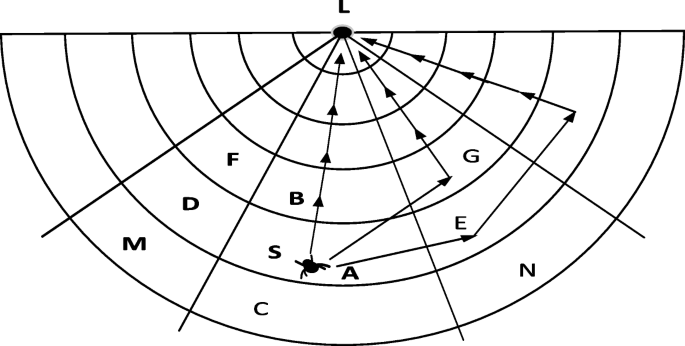Fig. 4

### Definition 5.

Chord-oriented region: If (ρm, ρm + 1, θn, θn + 1) refers to a small region A in the model, the mathematically expressed region with the same chord as A in the region adjacent to region A is called the chord-oriented region. As shown in Fig. 4, regions B and C both have the same chord ρm, ρm + 1 as A, so B and C are termed as the chord-oriented regions of A. According to Theorem 1, the mathematical expression of chord-oriented region B is (ρm − 1, ρm, θn, θn + 1), referred to as the chord-oriented meta-region where the polar angle decreases, and the mathematical expression of chord-oriented region C is (ρm + 1, ρm + 2, θn, θn + 1), referred to as the chord-oriented meta-region where the polar angle increases.

### Definition 6.

Radial-oriented region: If (ρm, ρm + 1, θn, θn + 1) refers to a small region A in the model, the expressed region with the same radial as A is called the radial-oriented region. As shown in Fig. 4, the regions D and E both have the same radial θn, θn + 1 as A, so D and E are termed as the radial-oriented regions of A. According to Theorem 1, the mathematical expression of radial-oriented region D is (ρm, ρm + 1, θn − 1, θn), referred to as the radial-oriented meta-region where the polar radius decreases, and the mathematical expression of radial-oriented region E is (ρm, ρm + 1, θn + 1, θn + 2), referred to as the radial-oriented meta-region where the polar radius increases.

### Definition 7.

Binary bit sequence chain: the binary bit sequence b0b1b2bn(bi = 0 or 1, i = 0, 1, n) is used to store the relevant information of the non-overlapping meta-region chain, where the first three b0b1b2 in b0b1b2bn express the meta-region where the chain head node is located, and every two elements starting from the fourth element express a meta-region.

When b2i − 1 = 1 (i ≥ 2), it indicates that the ith meta-region is the meta-region where the polar radius decreases or the polar angle decreases;

When b2i − 1 = 0 (i ≥ 2), it indicates that the ith meta-region is the meta-region where the polar radius increases or the polar angle increases;

When b2i = 0 (i ≥ 2), it indicates that the ith meta-region is the chord-oriented meta-region;

When b2i = 1 (i ≥ 2), it indicates that the ith meta-region is the radial-oriented meta-region.

Each binary bit sequence chain corresponds to a non-overlapping meta-region chain, and the next meta-region of the current non-overlapping meta-region chain can be calculated by formulas (6) and (7):

$${\rho}_{\mathrm{next}}={\rho}_x\mid x=m-{\sum}_{i=0}^p\left(2{b}_{2i+3}-1\right){b}_{2i+4}\kern14.75em$$
(6)
$${\theta}_{\mathrm{next}}={\theta}_y\mid y=n-{\sum}_{i=0}^p\left(2{b}_{2i+3}-1\right)\left(1-{b}_{2i+4}\right)$$
(7)

Where m and n are the pointers of the binary bit sequence chain.

According to formulas (6) and (7), the binary bit sequence chain can completely describe the non-overlapping meta-region chain and determine the region where any neighbor node of the current node is located.

## Results and discussion

### Disjoint multipath routing

According to the virtual spider network model with disjoint multipaths, the source node S can transmit data to the sink node along different paths of different non-overlapping meta-region chains. The packet format includes the storage source node S, sink node Sink, chain head meta-region selected by the current packet, binary bit sequence chain, bit sequence chain pointer, and data address in Table 1.

When an intermediate node receives a packet, it first updates the bit sequence chain pointer at the head of the packet and transmits data to the next-hop node on the basis of the dynamic next-hop selection mechanism. Figure 4 shows the three disjoint paths that may transmit data from the source node S to the sink node Sink. When a node in a certain area selects its next-hop node, the following three mechanisms can be adopted.

(1) Distance priority mechanism: The node to be selected has the minimum Euclidean distance from the node that is currently transmitting the packet, and the next-hop node that can become the current node needs to meet certain conditions.

In the region adjacent to the region where the current node is located;

A node in the neighbor node set of the current node;

A node with a minimum Euclidean distance from the current node. The minimum distance formula is defined as (8):

$$\mathrm{l}=\min \left\{\sqrt{{\rho_j}^2+{\rho}^2-2{\rho}_j\rho \cos \left(\theta -{\theta}_j\right)}|{\rho}_q<\rho <{\rho}_{q+1},{\theta}_p<\theta <{\theta}_{p+1}\right\}$$
(8)

Where (ρj, θj) is the coordinate of the neighbor node, (ρ, θ) is the coordinate of the current node, and (ρq, ρq + 1, θp, θp + 1) mathematically refers to the next region of the region where the current node is located.

(2) Angle priority mechanism: The neighbor node with the minimum deviation from the reference polar angle is preferentially selected as the next hop of the current node. The next-hop node that can become the current node needs to meet certain conditions.

A node in an adjacent area;

The polar radius of the region where the selected node is located cannot be larger than that of the current node;

The neighbor node with the minimum deviation from the reference polar angle is selected. The reference polar angle formula is defined as (9):

$${\theta}_{\mathrm{base}}=\frac{\theta_a+{\theta}_b}{2}$$
(9)

Where θa and θb are the two radials of the current region.

(3) Weight priority mechanism: The node with the minimum weight in the neighbor node set is preferentially selected as the next hop of the current node.

If the node P1 in region A is to transmit data information, it first sends a hello message to the surrounding nodes, and the node in region A does not respond to the message. But if the node in the region adjacent to region A receives the message, and it also needs to send a response to the node P1, which carries the distance from the node to the node P1 and the residual energy of the current node. Creating a dynamic neighbor node table, the node P1 receives the response information sent by neighbor nodes within a period of sending the hello message, and records it in its neighbor node table. The field of the neighbor node table contains the number of neighbor node, residual energy, the distance to the node, etc., and P1 no longer receives response information after a period of time, and then the weight of each neighbor node is calculated and the node with the minimum weight is selected as the next hop of the current node according to the weight formula. The next hop that can become the current node needs to meet certain conditions.

1. A node in an adjacent area;

2. The polar radius of the region where the selected node is located is less than or equal to that of the current

node;

1. In the neighbor node set, the node with more residual energy and shorter distance to the current node would take precedence except for the node that meets the above two conditions. The weight calculation formula (10) is described as follows:

$$\omega \left({P}_i\right)=\frac{d_i}{d_{\mathrm{max}}}+1-\frac{E_i}{E_{\mathrm{max}}}$$
(10)

Where di is the distance from neighbor node i to the current node, dmax is the maximum distance to the node in the neighbor node set, Ei is the current residual energy of node i, and Emax is the maximum value of the residual energy of all nodes in the neighbor node set. According to the weight formula, it can be calculated that the node with shorter distance to the current node and more residual energy has less weight.

### Node movement processing and path repair

Among the three dynamic next-hop node selection mechanisms, the next-hop node that meets the conditions can be found ideally to transmit data groupings. However, when the nodes in the network are unevenly distributed, or when the energy consumption is not balanced, it is possible the current node cannot find the next-hop node that meets the conditions, resulting in the break of links. This algorithm adopts an inter-regional “borrowing node” strategy to repair the link break. The “borrowing node” repair strategy steps are as follows:

(1) In the current Meta-region, Meta-region A and the eight adjacent non-overlapping meta-regions, including the middle upper (MU) region, middle down (MD) region, left middle (LM) region, right middle (RM) region, left upper (LU) region, right upper (RU) region, left down (LD) region, and right down (RD) region. According to (10), the node with the minimum weight is selected as the next-hop node and marked as “borrowed,” which is also called “borrowing node.”

(2) The region where the borrowing nodes (6) and (7) are located is modified as the next meta-region indicated by the bit sequence chain, a “borrowed” mark is added into the borrowing node, and the pointer of the bit sequence chain is increased by 1.

(3) The marked node cannot be used as a node in the original non-overlapping region during the borrowing period. After the node transmits the data, and its borrowed mark would be removed, it can only be used as a node in the normal region.

(4) During the borrowing period, the marked node cannot be used by other paths or repeatedly marked, and can only be borrowed by one path.

(5) After the borrowing period expires, the “borrowed” mark is fully removed and the node can be reused as a node in the original non-overlapping meta-region chain.

The “borrowing node” strategy can not only enable packets to be transmitted across meta-regions, but also ensure that the multipath is disjoint, thus effectively repairing link break.

### Simulation and analysis

DMRRD algorithm is compared with Energy and Mobility-aware Geographical Multipath Routing (EM-GMR) algorithm  and AGEM algorithm . The selected performance indexes include path failure rate, packet loss rate, and network lifetime. The simulation experiment is conducted in the following scenarios: Nodes in the network are randomly distributed in a square region 400 m × 400 m, the sink node is in the center of the region, the energy of the sink node is infinite, and a certain number of mobile nodes and fixed nodes are set in the region. The mobile node accounts for 50% of the total number of nodes, and the mobile node randomly selects an angle from [0, 2π] for each movement. When the number N of nodes is 260, the communication radius between nodes is 40 m, the data transmission speed is 160 kb/s, and the simulation environment is carried out under a MATLAB 2017 environment.

One of the three modes can be selected to find the next hop of the current node in the process of multi-path establishment. However, the selection of the next-hop node based on weight priority needs to broadcast information first, so its energy consumption is larger than the first two. Performance simulation experiment of the routing algorithm based on the three modes respectively are shown as follows. In the simulation, the models based on distance priority and angle priority are represented by DMRRD1, and the model based on weight priority is represented by DMRRD2.

As can be seen from Fig. 5, with the increase of node movement rate, the path failure rate of EM-GMR algorithm adds rapidly, followed by that of AGEM algorithm. The algorithm proposed herein has the smallest change, because the multipath routing of non-overlapping regions can well adapt to the node movement, while this algorithm has adopted favorable measures to deal with node movement and path failure. When the speed of node movement is slower, the weight-based mode has a lower path failure rate than the distance and angle-based modes, because the weight-based mode can use the energy of neighbor nodes in neighbor regions in a balanced way. As the speed of node movement increases, the weight-based mode needs to send hello information and receive response information every time it searches for the next-hop node, so that the energy consumption of the node is larger, and the path failure rate is higher than that of the angle- and distance-based modes. EM-GMR algorithm will consume numerous energy to reroute the path failure caused by node movement, and the path failure rate caused by node movement and energy depletion is relatively high. As can be seen from Fig. 6, when the number of nodes in the network is smaller, the path failure rate of the algorithm in this paper is lower than that of AGEM algorithm, because the nodes are fewer and uneven. If there is no node in the region adjacent to a region in the algorithm, it may lead to link failure. It can be seen that this algorithm is more applicable to networks with high node density.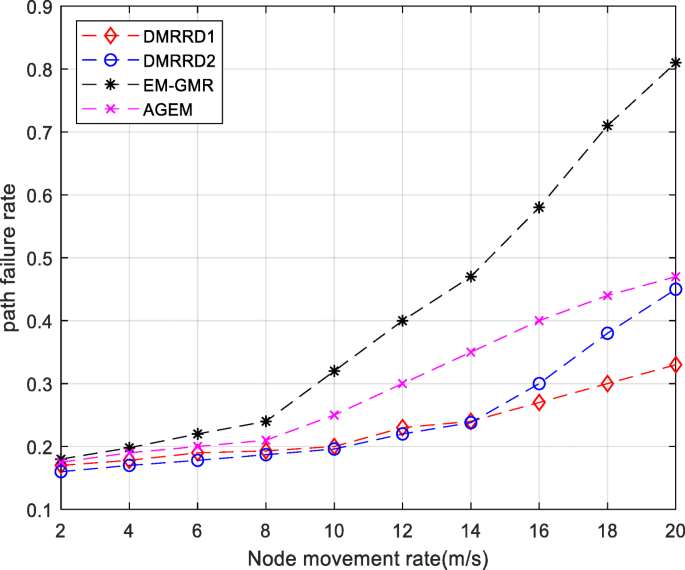Fig. 5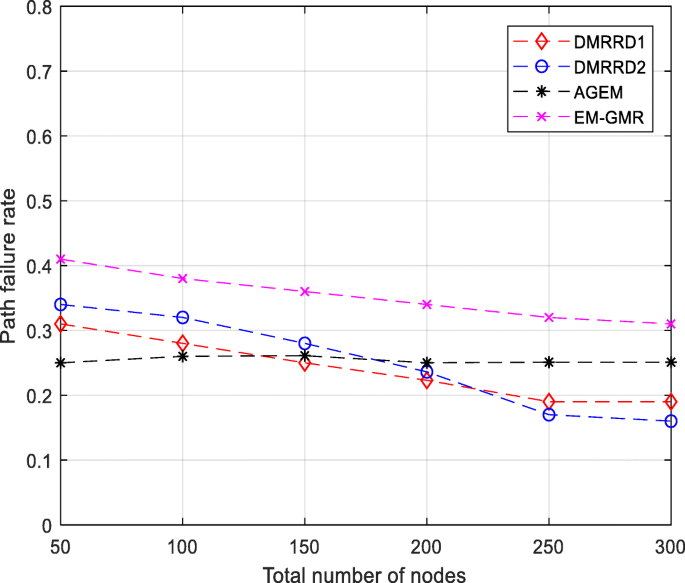Fig. 6

Experiments on the transmission accuracy of packets in the network have been carried out in Fig. 7 and Fig. 8. It can be seen that the packet loss rate increases slightly, when the speed of the mobile node in the algorithm proposed herein rises. By contrast, the packet loss rate of the AGEM algorithm adds rapidly when the speed of the mobile node exceeds 8 m/s. With the increase of the node number in the network, the packet loss rate of the algorithm in this paper, AGEM and EM-GMR algorithms, will decrease accordingly, but the algorithm proposed herein still has great advantages over the other two algorithms in packet loss rate.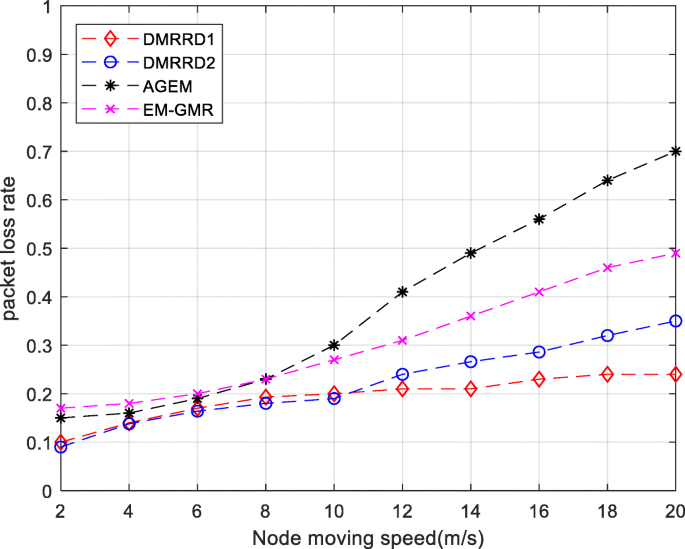Fig. 7Fig. 8

In Fig. 9 and Fig. 10, the algorithm proposed herein has a long network lifetime, when the node movement speed rises or when the number of nodes in the network increases. EM-GMR multipath algorithm needs to use a fuzzy logic system to make decisions when selecting the next-hop node. AGEM algorithm determines the forwarding of packets according to the node’s location information, so the path failure caused by node movement needs to reconstruct path, but frequent path reconstruction consumes numerous energy and the network lifetime performance is poor. It can be seen that the lifetime of the third mode is shorter than that of the first two modes because when the next-hop node is selected, the third mode needs to broadcast path creation information to neighbor regions first, which consumes more energy than the first two algorithms. In comparison with EM-GMR and AGEM protocols, the algorithm proposed herein only needs to maintain the neighbor node information, which consumes less energy.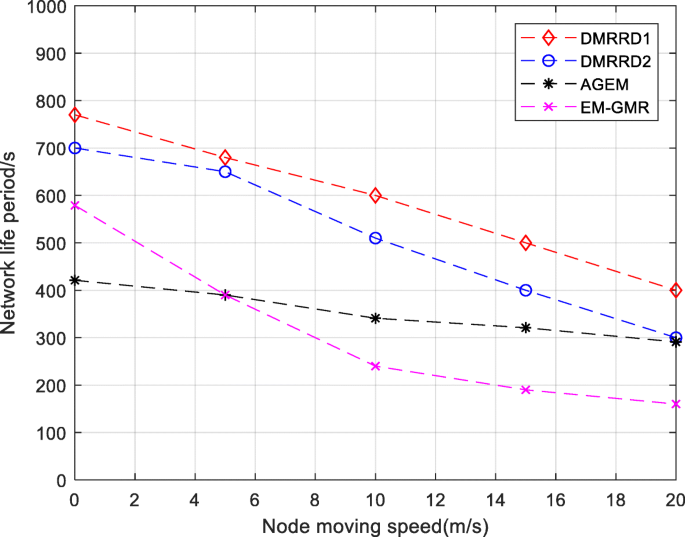Fig. 9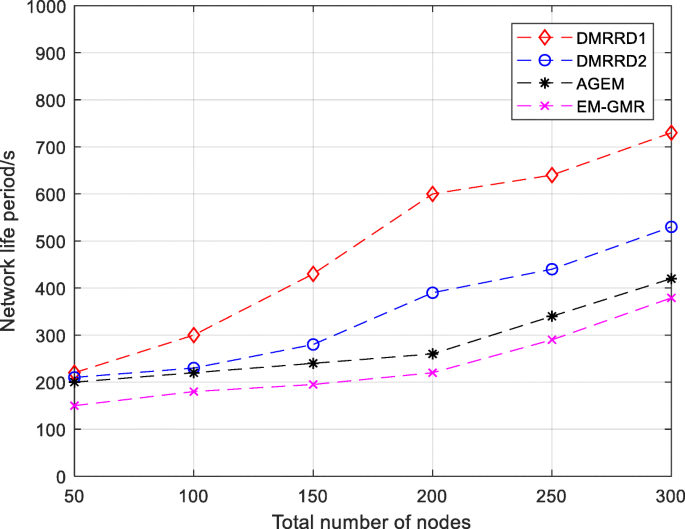Fig. 10

## Conclusion

Aiming at the topology change and “hot area” of WSN, this paper puts forward DMRRD algorithm and provides the region division model of the whole network, showing the selection procedures and specific ideas of disjoint multipath. In the process of path formation, three modes are proposed, respectively, based on distance priority, angle priority, and weight priority. The DMRRD algorithm is tested in a certain environment; the algorithm is particularly proposed for mobile nodes. The simulation process is divided into two conditions to compare and analyze the network performance. One is to compare and analyze the network performance by changing the rate of mobile nodes when the number of nodes in the network is constant, and the other is to do so when the total number of nodes in the network changes and the moving rate of nodes is constant. Experimental results show the algorithm can effectively prolong the lifetime of the network and boasts a lower packet loss rate and path failure rate.

## Abbreviations

AGEM:

An Energy Geographical Multipath Routing

DMRRD:

Disjoint multipath routing algorithm based on region division

DMRRD1:

Disjoint multipath routing algorithm based on distance priority and angle priority

DMRRD2:

Disjoint multipath routing algorithm based on weight priority

EM-GMR:

Energy and Mobility-aware Geographical Multipath Routing

WSN:

Wireless sensor networks

## References

1. 1.

M. Dasgupta, G.P. Biswas, Design of multi-path data routing algorithm based on network reliability [J]. Comput Electr Eng 38(6), 1433–1443 (2012)

2. 2.

F. Xiao, W. Liu, Z. Li, L. Chen, R. Wang, Noise-tolerant wireless sensor networks localization via multi-norms regularized matrix completion. IEEE Trans Veh Technol 67(3), 2409–2419 (2018)

3. 3.

H. Zhu, F. Xiao, L. Sun, R. Wang, P. Yang, R-TTWD: Robust device-free through-the-wall detection of moving human with WiFi. IEEE Journal on Selected Areas in Communications 35(5), 1090–1103 (2017)

4. 4.

F. Xiao, X. Xie, Z. Jiang, L. Sun, R. Wang, Utility-aware data transmission scheme for delay tolerant networks. Peer-to-Peer Networking and Applications 9(5), 936–944 (2016)

5. 5.

J. Park, S. Lee, S. Yoo, Time slot assignment for convergecast in wireless sensor networks[J]. Journal of Parallel & Distributed Computing 83, 70-82 (2015)

6. 6.

J. Song, Y. Miao, E. Song, et al., Reliability-aware cooperative node sleeping and clustering in duty-cycled sensors networks:[J]. Sensors 18(1), 127 (2018)

7. 7.

Q. Liang, Q. Ren, Energy and mobility aware geographical multipath routing for wireless sensor networks. IEEE Communications Society 3, 1867–1871 (2015)

8. 8.

P. Mérindol, P. Francois, O. Bonaventure, et al., An efficient algorithm to enable path diversity in link state routing networks. Comput Netw 55(5), 1132–1149 (2011)

9. 9.

E. Lee, S. Park, S. Oh, S.-H. Kim, Rendezvous-based data dissemination for supporting mobile sinks in multi-hop clustered wireless sensor networks. Wirel Netw 20(8), 2319–2336 (November 2014)

10. 10.

C. Wu, L. Wang, Y. Wang, A competition-based algorithm for routing discovery and repair in large-scale VANET [J]. Ksii Transactions on Internet & Information Systems 11(12), 5729–5744 (2017)

11. 11.

S. Medjiah, T. Ahmed, F.A.G.E.M. Krief, Adaptive greedy-compass energy-aware multipath routing protocol for WMSNs// proceedings of the 7th IEEE conference on consumer communications and networking conference. IEEE Press 40(6), 106–114 (2018)

12. 12.

A. Rana, A. Garg, S.K. Chaurasiy, An Enhanced Energy Aware Coverage Preservation with Static Clustering for WSN[J]. International Journal of Computer Applications 102(15), 38-41 (2014)

13. 13.

V.R. Budyal, S.S. Manvi, ANFIS and agent based bandwidth and delay aware anycast routing in mobile ad hoc networks. J Netw Comput Appl 39(3), 140–151 (2014)

Download references

## Acknowledgements

This research is sponsored by the National Natural Science Foundation of China under Grant No. 61571150 and 61502037, The Natural Science Foundation of Heilongjiang Province of China under Grant No. F2017029, and Heilongjiang Provincial Education Office Project (135109237) and (135209235), Postgraduate Innovative Research Projects YJSCX2018-ZD09.

Not applicable.

## Author information

Authors

### Contributions

LSW is the main writer of this paper. He proposed the main idea, deduced the performance of the creation of virtual spider web model, completed the simulation, and analyzed the result. XCH introduced the DMRRD1 algorithm. YG gave some important suggestions for DMRRD2. All authors read and approved the final manuscript.

### Corresponding author

Correspondence to Lian-suo Wei.

## Ethics declarations

### Competing interests

The authors declare that they have no competing interests.

### Publisher’s Note

Springer Nature remains neutral with regard to jurisdictional claims in published maps and institutional affiliations.

## Rights and permissions

Open Access This article is distributed under the terms of the Creative Commons Attribution 4.0 International License (http://creativecommons.org/licenses/by/4.0/), which permits unrestricted use, distribution, and reproduction in any medium, provided you give appropriate credit to the original author(s) and the source, provide a link to the Creative Commons license, and indicate if changes were made.

Reprints and Permissions